# KSEEB Solutions for Class 9 Maths Chapter 14 Statistics Ex 14.4

In this chapter, we provide KSEEB SSLC Class 9 Maths Chapter 14 Statistics Ex 14.4 for English medium students, Which will very helpful for every student in their exams. Students can download the latest KSEEB SSLC Class 9 Maths Chapter 14 Statistics Ex 14.4 pdf, free KSEEB SSLC Class 9 Maths Chapter 14 Statistics Ex 14.4 pdf download. Now you will get step by step solution to each question.

## Karnataka Board Class 9 Maths Chapter 14 Statistics Ex 14.4

Question 1.
The following number of goals were scored by a team in a series of 10 matches :
2, 3, 4, 5, 0, 1. 3, 3, 4, 3
Find the mean, median and mode of these scores.
Solution:
Number of goals scored by a team:
(i) 2, 3, 4, 5, 0, 1, 3, 3, 4, 3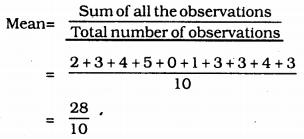= 2.8

(ii) If number of goals are arranged in ascending order,
0, 1, 2, 3, 3. 3, 3, 4, 4. 5
No. of observations =10 (even number)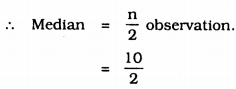= 5th observation.
= 3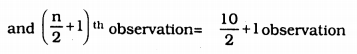= 5 + 1
= 6th observation ➝ 3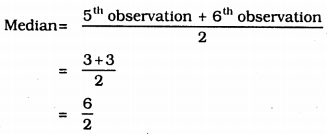= 3

(iii) Mode is the value of the observation which occurs most frequently.
Here 3 is repeated 4 times.
∴ Mode = 3.

Question 2.
In a mathematics test given to 15 students, the following marks (out of 100) are recorded : 41, 39, 48, 52, 46, 62, 54, 40, 96, 52, 98, 40, 42, 52, 60
Find the mean, median and mode of this data.
Solution:
In a mathematics test given to 15 students, the following marks (out of 100) are recorded :
41, 39, 48, 52, 46, 62, 54, 40, 96, 52, 98, 40, 42, 52, 60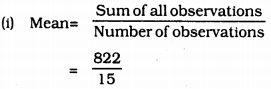Mode= 54.8

(ii) When marks are arranged in ascending order,
39, 40, 40, 41, 42, 46, 48, 52, 52, 52, 54, 60, 62, 96, 98
Number of observations = 15 (odd number)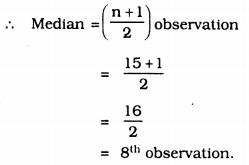∴ Median = 52.

(iii) Mode: It is the repeated value.
Here 52 is repeated three times.
∴ Mode = 52.

Question 3.
The following observations have been arranged in ascending order. If the median of the data is 63. find the value of x,
29, 32, 48, 50, x, x + 2, 72, 78, 84, 95
Solution:
29, 32, 48, 50, x, x + 2, 72, 78, 84, 95
Median = 63.
Number of observations = 10 (even number)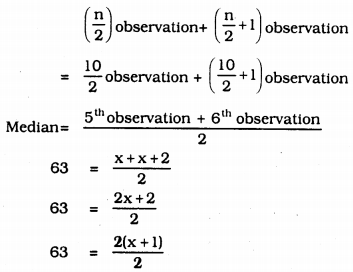∴ x + 1 = 63 ∴ x = 63 – 1
∴ x = 62.

Question 4.
Find the mode of 14, 25, 14, 28, 18, 17, 18, 14, 23, 22, 14, 18.
Solution:
14, 25, 14, 28, 18, 17, 18, 14, 23, 22; 14, 18
Mode = ?
Arranging the scores in ascending order,
14, 14, 14, 14, 17, 18, 18, 18, 22, 23, 25, 28
Mode is the repeated value.
∴ Here 14 is repeated 4 times.
∴ Mode = 14.

Question 5.
Find the mean salary of 60 workers of a factory from the following table :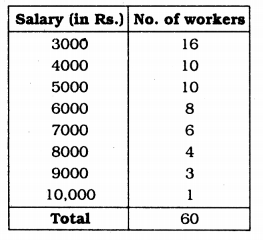Solution: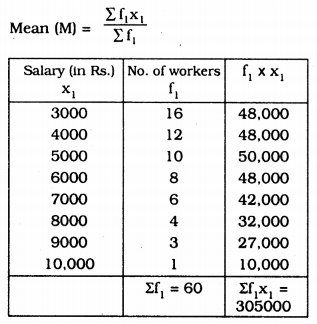∴ The men salary of workers is Rs. 5083.33.

Question 6.
Give one example of a situation in which
(i) The mean is an appropriate measure of central tendency.
(ii) The mean is not an appropriate measure of central tendency but the median is appropriate of central tendency.
Solution:
(i) Each score is in lesser difference, it is easy to calculate the average than the median.
Ex: monthly salary of 5 persons, 10000, 10100, 10200, 10300, 10400
Mean of the scores is 10200.

(ii) If scores have more differences, it is easy to calculate the median than the mean.
Ex.: Marks obtained by 7 students in Mathematics :
2, 10, 20, 15, 4, 23, 3
Ascending Order : 2, 3, 4, 10, 15, 20, 23
Median =10 but the mean is 11.

All Chapter KSEEB Solutions For Class 9 Maths

—————————————————————————–

All Subject KSEEB Solutions For Class 9

*************************************************

I think you got complete solutions for this chapter. If You have any queries regarding this chapter, please comment on the below section our subject teacher will answer you. We tried our best to give complete solutions so you got good marks in your exam.

If these solutions have helped you, you can also share kseebsolutionsfor.com to your friends.

Best of Luck!!